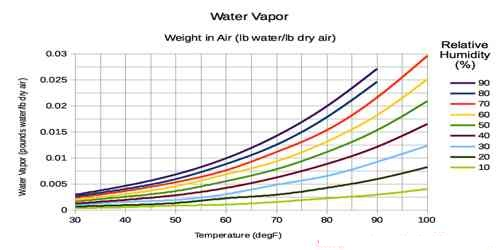Chemistry

# Relation between Water Vapour and Air PressureRelation between water vapour and air pressure

We know, if there is water vapour or if air is moist then its density also changes. Density of moist or vapour containing water vapour is less than that of dry air. That means, as the water vapour increases in air, its density also decreases.

In atmosphere there always exists some water vapour. Through evaporation process enough water are evaporated from canals, ponds, water reservoir, rivers, oceans etc and mixes with atmosphere. From cloud, rain, fog, dew etc natural phenomena it is proved that there is enough water vapour in air.

Amount of water vapour in atmosphere depends on the sources of water, latitude, location of the place above the sea level etc.

Suppose, air of mass in at pressure P1 and temperature T1K, has volume of V1 and density ofρ1. and at pressure P2 and temperature T2K if the volume of that air is V2 and density is ρ2, then

ρ1 = m/V1  or, V1 = m/ρ1

and, ρ2 = m/2  or, V2 = m/ρ2

Now, inserting the values of V1 and V2 in the relation.

P1V1/T1 = P2V2/T2

we get,

P1m/ρ1T1 = P2m/ρ2T2 = Constant

or, P11T1 = P22T2 = Constant

or, ρ1T1/ P1 = ρ2T2/P2 = Constant …. ….. (1)

then, ρT/P = Constant

This equation gives the relation between pressure and density of water vapour at temperature T. If temperature remains constant, i.e., if, T1 = T2 then from equation (1)

we get, ρ1/P1 = ρ2/P2 = constant.

or, ρ/P = constant

so, ρ ∞ P